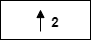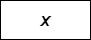Main Content

# idwt

Single-level inverse discrete 1-D wavelet transform

## Syntax

``x = idwt(cA,cD,wname)``
``x = idwt(cA,cD,LoR,HiR)``
``x = idwt(___,l)``
``x = idwt(___,'mode',mode)``
``x = idwt(cA,[],___)``
``x = idwt([],cD,___)``

## Description

example

````x = idwt(cA,cD,wname)` returns the single-level one-dimensional wavelet reconstruction `x` based on the approximation and detail coefficients `cA` and `cD`, respectively, using the wavelet specified by `wname`. For more information, see `dwt`.Let `la` be the length of `cA` (which also equals the length of `cD`), and `lf` the length of the reconstruction filters associated with `wname` (see `wfilters`). If the DWT extension mode is set to periodization, then the length of `x` is equal to 2`la`. Otherwise, the length of `x` is equal to 2`la`- 2`lf`+2. For more information, see `dwtmode`.```

example

````x = idwt(cA,cD,LoR,HiR)` uses the specified lowpass and highpass wavelet reconstruction filters `LoR` and `HiR`, respectively.```
````x = idwt(___,l)` returns the length-`l` central portion of the reconstruction. This argument can be added to any of the previous input syntaxes```
````x = idwt(___,'mode',mode)` uses the specified DWT extension mode `mode`. For more information, see `dwtmode`. This argument can be added to any of the previous syntaxes.```
````x = idwt(cA,[],___)` returns the single-level reconstructed approximation coefficients based on the approximation coefficients `cA`.```
````x = idwt([],cD,___)` returns the single-level reconstructed detail coefficients based on the detail coefficients `cD`.```

## Examples

collapse all

Demonstrate perfect reconstruction using `dwt` and `idwt` with an orthonormal wavelet.

```load noisdopp; [A,D] = dwt(noisdopp,'sym4'); x = idwt(A,D,'sym4'); max(abs(noisdopp-x))```
```ans = 3.2156e-12 ```

Demonstrate perfect reconstruction using `dwt` and `idwt` with a biorthogonal wavelet.

```load noisdopp; [Lo_D,Hi_D,Lo_R,Hi_R] = wfilters('bior3.5'); [A,D] = dwt(noisdopp,Lo_D,Hi_D); x = idwt(A,D,Lo_R,Hi_R); max(abs(noisdopp-x))```
```ans = 3.5527e-15 ```

## Input Arguments

collapse all

Approximation coefficients, specified as a vector. `cA` is expected to be the output of `dwt`.

Data Types: `single` | `double`
Complex Number Support: Yes

Detail coefficients, specified as a vector. `cD` is expected to be the output of `dwt`.

Data Types: `single` | `double`
Complex Number Support: Yes

Wavelet used to compute the single-level inverse discrete wavelet transform (IDWT), specified as a character vector or string scalar. The wavelet must be recognized by `wavemngr`. The wavelet is from one of the following wavelet families: Daubechies, Coiflets, Symlets, Fejér-Korovkin, Discrete Meyer, Biorthogonal, and Reverse Biorthogonal. See `wfilters` for the wavelets available in each family.

The wavelet specified must be the same wavelet used to obtain the approximation and detail coefficients.

Example: `'db4'`

Wavelet reconstruction filters, specified as a pair of even-length real-valued vectors. `LoR` is the lowpass reconstruction filter, and `HiR` is the highpass reconstruction filter. The lengths of `LoR` and `HiR` must be equal. See `wfilters` for additional information.

Data Types: `single` | `double`

Length of central portion of reconstruction, specified as a positive integer. If `xrec = idwt(cA,cD,wname)`, then `l` cannot exceed `length(xrec)`.

Data Types: `single` | `double`

DWT extension mode used in the wavelet reconstruction, specified as a character vector or string scalar. For possible extension modes, see `dwtmode`.

## Algorithms

Starting from the approximation and detail coefficients at level j, cAj and cDj, the inverse discrete wavelet transform reconstructs cAj−1, inverting the decomposition step by inserting zeros and convolving the results with the reconstruction filters.where

•— Insert zeros at even-indexed elements

•— Convolve with filter X

•— Take the central part of U with the convenient length

 Daubechies, I. Ten Lectures on Wavelets. CBMS-NSF Regional Conference Series in Applied Mathematics. Philadelphia, PA: Society for Industrial and Applied Mathematics, 1992.

 Mallat, S. G. “A Theory for Multiresolution Signal Decomposition: The Wavelet Representation.” IEEE Transactions on Pattern Analysis and Machine Intelligence. Vol. 11, Issue 7, July 1989, pp. 674–693.

 Meyer, Y. Wavelets and Operators. Translated by D. H. Salinger. Cambridge, UK: Cambridge University Press, 1995.

## Support#### Try MATLAB, Simulink, and Other Products

Get trial now